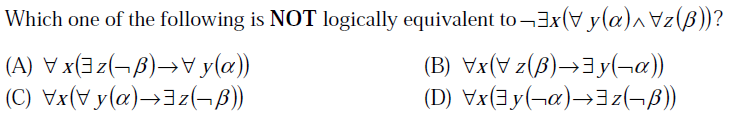GFG App
Open AppBrowser
Continue

# GATE | GATE CS 2013 | Question 47(A) A
(B) B
(C) C
(D) D

Explanation:

Given statement is :

¬ ∃ x ( ∀y(α) ∧ ∀z(β) )

```where ¬ is a negation operator, ∃ is Existential Quantifier with the
meaning of "there Exists", and ∀ is a Universal Quantifier
with the meaning   " for all " , and α, β can be treated as predicates.

here we can apply some of the standard
results of Propositional and 1st order logic on the given statement,
which are as follows :

[ Result 1 : ¬(∀x P(x)) <=> ∃ x¬P(x), i.e. negation
of "for all" gives "there exists" and negation also gets applied to scope of
quantifier, which is P(x) here. And also negation of "there exists" gives "for all",
and negation also gets applied to scope of quantifier  ]

[ Result 2 :  ¬ ( A ∧ B ) = ( ¬A  ∨ ¬B )  ]

[ Result 3 :  ¬P  ∨ Q <=> P -> Q ]

[ Result 4 : If P ->Q, then by Result of Contrapositive,  ¬Q -> ¬P  ]

```

Now we need to use these results as shown below:

```

¬ ∃ x ( ∀y(α) ∧ ∀z(β) )                 [ Given ]

=> ∀ x (¬∀y(α) ∨ ¬∀z(β) )          [ after applying Result 1 & Result 2 ]

=> ∀ x ( ∀y(α) -> ¬∀z(β) )     [after applying Result 3 ]

=> ∀ x ( ∀y(α) -> ∃z(¬β) )      [after applying Result 1]

which is same as the statement C.

Hence the Given Statement is logically Equivalent
to the statement C.

Now, we can also prove that given statement is logically equivalent to the statement
in option  B.

Let's see how !

The above derived statement is :

∀ x ( ∀y(α) -> ∃z(¬β) )

Now this statement can be written as (or equivalent to) :

=> ∀ x ( ∀z(β) -> ∃y(¬α) )     [after applying Result 4 ]

And this statement is same as statement B.
Hence the Given statement is also logically equivalent
to the statement B.

So, we can conclude that the Given statement is NOT logically equivalent to the
statements A and D.
```

Hence, the correct answer is Option A and Option D. But in GATE 2013, marks
were given to all for this question.

Quiz of this Question

My Personal Notes arrow_drop_up